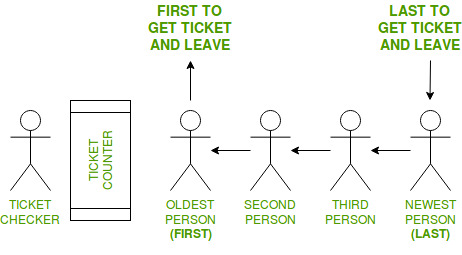Open in App
Not now

# FIFO (First-In-First-Out) approach in Programming

• Difficulty Level : Basic
• Last Updated : 06 Dec, 2022

FIFO is an abbreviation for first in, first out. It is a method for handling data structures where the first element is processed first and the newest element is processed last.

Real-life example:In this example, following things are to be considered:

• There is a ticket counter where people come, take tickets and go.
• People enter a line (queue) to get to the Ticket Counter in an organized manner.
• The person to enter the queue first, will get the ticket first and leave the queue.
• The person entering the queue next will get the ticket after the person in front of him
• In this way, the person entering the queue last will the tickets last
• Therefore, the First person to enter the queue gets the ticket first and the Last person to enter the queue gets the ticket last.

This is known as First-In-First-Out approach or FIFO.

Where is FIFO used:

1. Data Structures:
• Certain data structures like Queue and other variants of Queue uses FIFO approach for processing data.
2. Disk scheduling:
• Disk controllers can use the FIFO as a disk scheduling algorithm to determine the order in which to service disk I/O requests.
3. Communications and networking”
• Communication network bridges, switches and routers used in computer networks use FIFOs to hold data packets en route to their next destination.

Program Examples for FIFO

Program 1: Queue

## C++

 `// C++ program to demonstrate ` `// working of FIFO ` `// using Queue interface in C++`   `#include` `using` `namespace` `std;`   `// print the elements of queue` `void` `print_queue(queue<``int``> q)` `{` `    ``while` `(!q.empty())` `    ``{` `        ``cout << q.front() << ``" "``;` `        ``q.pop();` `    ``}` `    ``cout << endl;` `}`   `// Driver code` `int` `main() ` `{ ` `    ``queue<``int``> q ;`   `    ``// Adds elements {0, 1, 2, 3, 4} to queue ` `    ``for` `(``int` `i = 0; i < 5; i++) ` `        ``q.push(i); `   `    ``// Display contents of the queue. ` `    ``cout << ``"Elements of queue-"``;` `        `  `    ``print_queue(q);`   `    ``// To remove the head of queue. ` `    ``// In this the oldest element '0' will be removed ` `    ``int` `removedele = q.front();` `    ``q.pop();` `    ``cout << ``"removed element-"` `<< removedele << endl; `   `    ``print_queue(q);`   `    ``// To view the head of queue ` `    ``int` `head = q.front(); ` `    ``cout << ``"head of queue-"` `<< head << endl; `   `    ``// Rest all methods of collection interface, ` `    ``// Like size and contains can be used with this ` `    ``// implementation. ` `    ``int` `size = q.size(); ` `    ``cout << ``"Size of queue-"` `<< size;` `        `  `    ``return` `0;` `} `   `// This code is contributed by Arnab Kundu`

## Java

 `// Java program to demonstrate` `// working of FIFO` `// using Queue interface in Java`   `import` `java.util.LinkedList;` `import` `java.util.Queue;`   `public` `class` `QueueExample {` `    ``public` `static` `void` `main(String[] args)` `    ``{` `        ``Queue q = ``new` `LinkedList<>();`   `        ``// Adds elements {0, 1, 2, 3, 4} to queue` `        ``for` `(``int` `i = ``0``; i < ``5``; i++)` `            ``q.add(i);`   `        ``// Display contents of the queue.` `        ``System.out.println(``"Elements of queue-"` `+ q);`   `        ``// To remove the head of queue.` `        ``// In this the oldest element '0' will be removed` `        ``int` `removedele = q.remove();` `        ``System.out.println(``"removed element-"` `+ removedele);`   `        ``System.out.println(q);`   `        ``// To view the head of queue` `        ``int` `head = q.peek();` `        ``System.out.println(``"head of queue-"` `+ head);`   `        ``// Rest all methods of collection interface,` `        ``// Like size and contains can be used with this` `        ``// implementation.` `        ``int` `size = q.size();` `        ``System.out.println(``"Size of queue-"` `+ size);` `    ``}` `}`

## Python3

 `# Python program to demonstrate` `# working of FIFO` `# using Queue interface in Python`   `q ``=` `[]`   `# Adds elements {0, 1, 2, 3, 4} to queue` `for` `i ``in` `range``(``5``):` `    ``q.append(i)`   `# Display contents of the queue.` `print``(``"Elements of queue-"` `, q)`   `# To remove the head of queue.` `# In this the oldest element '0' will be removed` `removedele ``=` `q.pop(``0``)` `print``(``"removed element-"` `, removedele)`   `print``(q)`   `# To view the head of queue` `head ``=` `q[``0``]` `print``(``"head of queue-"` `, head)`   `# Rest all methods of collection interface,` `# Like size and contains can be used with this` `# implementation.` `size ``=` `len``(q)` `print``(``"Size of queue-"` `, size)`   `# This code is contributed by patel2127.`

## C#

 `// C# program to demonstrate ` `// working of FIFO ` `using` `System;` `using` `System.Collections.Generic;`   `public` `class` `QueueExample ` `{ ` `    ``public` `static` `void` `Main(String[] args) ` `    ``{ ` `        ``Queue<``int``> q = ``new` `Queue<``int``>(); `   `        ``// Adds elements {0, 1, 2, 3, 4} to queue ` `        ``for` `(``int` `i = 0; i < 5; i++) ` `            ``q.Enqueue(i); `   `        ``// Display contents of the queue. ` `        ``Console.Write(``"Elements of queue-"``); ` `        ``foreach``(``int` `s ``in` `q) ` `                ``Console.Write(s + ``" "``); `   `        ``// To remove the head of queue. ` `        ``// In this the oldest element '0' will be removed ` `        ``int` `removedele = q.Dequeue(); ` `        ``Console.Write(``"\nremoved element-"` `+ removedele + ``"\n"``); ` `        ``foreach``(``int` `s ``in` `q) ` `                ``Console.Write(s + ``" "``); `   `        ``// To view the head of queue ` `        ``int` `head = q.Peek(); ` `        ``Console.Write(``"\nhead of queue-"` `+ head); `   `        ``// Rest all methods of collection interface, ` `        ``// Like size and contains can be used with this ` `        ``// implementation. ` `        ``int` `size = q.Count; ` `        ``Console.WriteLine(``"\nSize of queue-"` `+ size); ` `    ``} ` `} `   `// This code has been contributed by 29AjayKumar`

## Javascript

 ``

Output

```Elements of queue-0 1 2 3 4
removed element-0
1 2 3 4